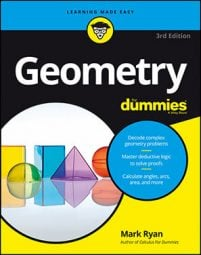##### Geometry For DummiesWith the side-splitter theorem, you draw one parallel line that divides a triangle's sides proportionally. With the extension of this theorem, you can draw any number of parallel lines that cut any lines (not just a triangle's sides) proportionally.

Extension of the Side-Splitter Theorem: If three or more parallel lines are intersected by two or more transversals, the parallel lines divide the transversals proportionally.

The following figure helps to illustrate this.

Given that the horizontal lines are parallel, the following proportions (among others) follow from the theorem:

Ready for a problem? Here goes:

Here's the proof diagram.

This is a long process, so you should go through the unknown lengths one by one.

1. Set up a proportion to get CD.

2. Now just subtract CD from BD to get BC.

3. Skip over the segments that make up segment FJ for a minute and use a proportion to find KL.

4. Subtract to get LM.

5. To solve for the parts of line FJ, use the total length of line FJ and the lengths along line AD.

To get FG, GH, and HJ, note that because the ratio AB : BC : CD is 12 : 24 : 8, which reduces to 3 : 6 : 2, the ratio of FG : GH : HJ must also equal 3 : 6 : 2. So let FG = 3x, GH = 6x, and HJ = 2x. Because you're given the length of line FJ, you know that these three segments must add up to 33:

A veritable walk in the park.